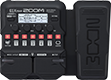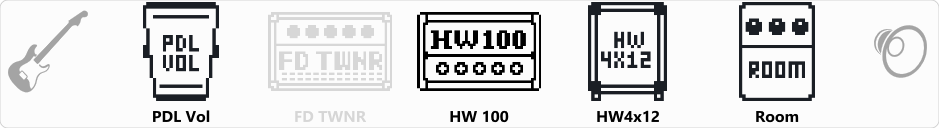# 21 Guns Acoustic

Discussion in 'Zoom G1/G1X Four' started by Swapnil, Oct 6, 2019.

1. 21 Guns AcousticDevice: Zoom G1X Four
Firmware: 1.10

Name on device: 21 intro
Optimized for: Guitar Amp

Effects chain:21 guns Acoustic

Effect: "PDL Vol" (Pedal), active - "yes"
"Volume" = 100
"Min" = 0
"Max" = 100
"Curve" = A

Effect: "FD TWNR" (Amp simulator), active - "no"
"Bass" = 48
"Middle" = 50
"Treble" = 45
"Bright" = OFF
"Gain" = 40
"Volume" = 80
"Depth" = 10
"Speed" = 50

Effect: "HW 100" (Amp simulator), active - "yes"
"Input" = BRILL
"Bass" = 40
"Middle" = 80
"Treble" = 60
"Presence" = 60
"Gain" = 50
"Volume" = 60

Effect: "HW4x12" (Cabinet), active - "yes"
"MIC" = ON
"D57: D421" = 50
"Hi" = 58
"Lo" = 50

Effect: "Room" (Reverb), active - "yes"
"PreD" = 28
"Decay" = 12
"Mix" = 38
"Tail" = On

Patch Volume: 28